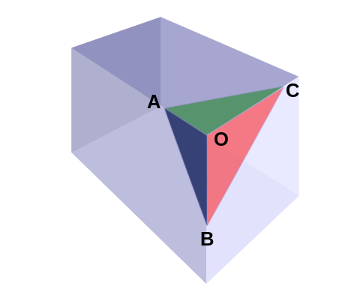# De Gua’s TheoremFrench mathematician Jean Paul de Gua de Malves discovered this three-dimensional analogue of the Pythagorean theorem in the 18th century.

If a tetrahedron has a right-angled corner (such as the corner of a cube), then the square of the area of the face opposite that corner is the sum of the squares of the areas of the other three faces.

Above,$A_{ABC}^{2} = A_{ABO}^{2} + A_{ACO}^{2} + A_{BCO}^{2}$